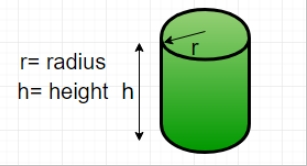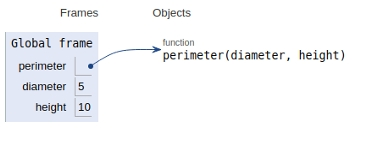# Find the perimeter of a cylinder in Python Program

PythonServer Side ProgrammingProgramming

## Problem statement

Input diameter and height, find the perimeter of a cylinder.

Perimeter is nothing but the side view of a cylinder i.e. a rectangle.

Therefore Perimeter= 2 * ( h + d )

here d is the diameter of the cylinder

h is the height of the cylinderNow let’s see the implementation

## Example

Live Demo

# Function to calculate the perimeter of a cylinder
def perimeter( diameter, height ) :
return 2 * ( diameter + height )
# main
diameter = 5 ;
height = 10 ;
print ("Perimeter = ",perimeter(diameter, height))

## Output

('Perimeter =', 30)

All the variables and functions are declared in the global frame as shown in the figure below.## Conclusion

In this article, we learned about finding the perimeter of a cylinder in Python 3.x. Or earlier.